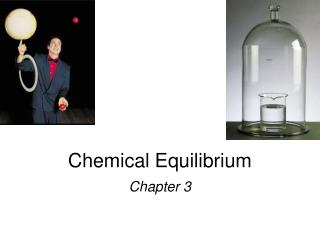Download PresentationChemical EquilibriumChemical Equilibrium - PowerPoint PPT Presentation

Download PresentationChemical Equilibrium
An Image/Link below is provided (as is) to download presentation

Download Policy: Content on the Website is provided to you AS IS for your information and personal use and may not be sold / licensed / shared on other websites without getting consent from its author. While downloading, if for some reason you are not able to download a presentation, the publisher may have deleted the file from their server.

- - - - - - - - - - - - - - - - - - - - - - - - - - - E N D - - - - - - - - - - - - - - - - - - - - - - - - - - -
Presentation Transcript

1. Chemical Equilibrium Chapter 3

2. H2O (l) H2O (g) N2O4(g) 2NO2(g) Equilibrium is a state in which there are no observable changes as time goes by. • Chemical equilibrium is achieved when: • the rates of the forward and reverse reactions are equal and • the concentrations of the reactants and products remain constant Physical equilibrium Chemical equilibrium 15.1

4. constant 15.1

5. N2O4(g) 2NO2(g) K = aA + bB cC + dD [NO2]2 [C]c[D]d [N2O4] K = [A]a[B]b = 4.63 x 10-3 Law of Mass Action 15.1

6. aA + bB cC + dD [C]c[D]d K = [A]a[B]b Equilibrium Will K >> 1 Lie to the right Favor products K << 1 Lie to the left Favor reactants 15.1

7. N2O4(g) 2NO2(g) Kc = Kp = In most cases Kc Kp P2 [NO2]2 NO2 [N2O4] aA (g) + bB (g)cC (g) + dD (g) P N2O4 Homogenous equilibrium applies to reactions in which all reacting species are in the same phase. Kp = Kc(RT)Dn Dn = moles of gaseous products – moles of gaseous reactants = (c + d) – (a + b) 15.2

8. CH3COOH (aq) + H2O (l) CH3COO-(aq) + H3O+ (aq) ‘ ‘ Kc = Kc [H2O] [CH3COO-][H3O+] [CH3COO-][H3O+] = [CH3COOH][H2O] [CH3COOH] Homogeneous Equilibrium [H2O] = constant Kc = 15.2

9. The equilibrium concentrations for the reaction between carbon monoxide and molecular chlorine to form COCl2 (g) at 740C are [CO] = 0.012 M, [Cl2] = 0.054 M, and [COCl2] = 0.14 M. Calculate the equilibrium constants Kc and Kp. CO (g) + Cl2(g) COCl2(g) = 0.14 0.012 x 0.054 [COCl2] [CO][Cl2] = 220 Kc= Kp = Kc(RT)Dn Dn = 1 – 2 = -1 R = 0.0821 T = 273 + 74 = 347 K Kp= 220 x (0.0821 x 347)-1 = 7.7 15.2

10. The equilibrium constant Kp for the reaction is 158 at 1000K. What is the equilibrium pressure of O2 if the PNO = 0.400 atm and PNO = 0.270 atm? 2 PNO PO = Kp 2 PNO 2 Kp = 2NO2 (g) 2NO (g) + O2 (g) PO PO = 158 x (0.400)2/(0.270)2 2 2 2 2 PNO PNO 2 2 2 = 347 atm 15.2

11. CaCO3(s) CaO (s) + CO2(g) ‘ ‘ Kc = Kc x [CaO][CO2] [CaCO3] [CaCO3] [CaO] Kp = PCO 2 Heterogenous equilibrium applies to reactions in which reactants and products are in different phases. [CaCO3] = constant [CaO] = constant Kc= [CO2] = 15.2

12. CaCO3(s) CaO (s) + CO2(g) PCO PCO 2 2 does not depend on the amount of CaCO3 or CaO = Kp 15.2

13. Consider the following equilibrium at 295 K: The partial pressure of each gas is 0.265 atm. Calculate Kp and Kcfor the reaction? Kp = P NH3 NH4HS (s) NH3(g) + H2S (g) P H2S = 0.265 x 0.265 = 0.0702 Kp = Kc(RT)Dn Kc= Kp(RT)-Dn Dn = 2 – 0 = 2 T = 295 K Kc = 0.0702 x (0.0821 x 295)-2 = 1.20 x 10-4 15.2

14. The reaction quotient (Qc)is calculated by substituting the initial concentrations of the reactants and products into the equilibrium constant (Kc) expression. • IF • Qc > Kcsystem proceeds from right to left to reach equilibrium • Qc = Kc the system is at equilibrium • Qc < Kcsystem proceeds from left to right to reach equilibrium 15.4

15. N2(g) + 3H2(g) 2NH3(g) Equilibrium shifts left to offset stress Add NH3 Le Châtelier’s Principle If an external stress is applied to a system at equilibrium, the system adjusts in such a way that the stress is partially offset as the system reaches a new equilibrium position. 1- Changes in Concentration 15.5

16. Remove Remove Add Add aA + bB cC + dD Le Châtelier’s Principle 2-Changes in Concentration continued Change Shifts the Equilibrium Increase concentration of product(s) left Decrease concentration of product(s) right Increase concentration of reactant(s) right Decrease concentration of reactant(s) left 15.5

17. A (g) + B (g) C (g) Le Châtelier’s Principle 3- Changes in Volume and Pressure Change Shifts the Equilibrium Increase pressure Side with fewest moles of gas Decrease pressure Side with most moles of gas Increase volume Side with most moles of gas Decrease volume Side with fewest moles of gas 15.5

18. Le Châtelier’s Principle 4-Changes in Temperature Change Exothermic Rx Endothermic Rx Increase temperature K decreases K increases Decrease temperature K increases K decreases hotter colder 15.5

19. uncatalyzed catalyzed Le Châtelier’s Principle • 5- Adding a Catalyst • does not change K • does not shift the position of an equilibrium system • system will reach equilibrium sooner Catalyst lowers Ea for both forward and reverse reactions. Catalyst does not change equilibrium constant or shift equilibrium. 15.5

20. Change Equilibrium Constant Le Châtelier’s Principle Change Shift Equilibrium Concentration yes no Pressure yes no Volume yes no Temperature yes yes Catalyst no no 15.5

21. Home work 1-Give me the meaning of the following term: Equilibrium - Homogenous equilibrium - Heterogenous equilibrium